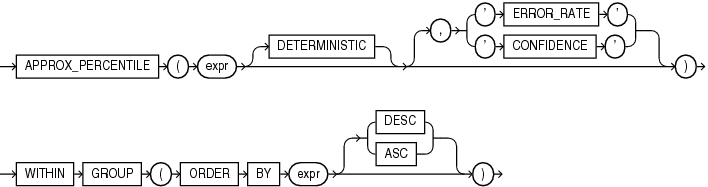## APPROX_PERCENTILE

SyntaxDescription of the illustration approx_percentile.eps

Purpose

`APPROX_PERCENTILE` is an approximate inverse distribution function. It takes a percentile value and a sort specification, and returns the value that would fall into that percentile value with respect to the sort specification. Nulls are ignored in the calculation

This function provides an alternative to the `PERCENTILE_CONT` and `PERCENTILE_DISC` functions, which returns the exact results. `APPROX_PERCENTILE` processes large amounts of data significantly faster than `PERCENTILE_CONT` and `PERCENTILE_DISC`, with negligible deviation from the exact result.

The first `expr` is the percentile value, which must evaluate to a numeric value between 0 and 1.

The second `expr`, which is part of the `ORDER` `BY` clause, is a single expression over which this function calculates the result. The acceptable data types for `expr`, and the return value data type for this function, depend on the algorithm that you specify with the `DETERMINISTIC` clause.

DETERMINISTIC

This clause lets you specify the type of algorithm this function uses to calculate the return value.

• If you specify `DETERMINISTIC`, then this function calculates a deterministic result. In this case, the `ORDER` `BY` clause expression must evaluate to a numeric value, or to a value that can be implicitly converted to a numeric value, in the range -2,147,483,648 through 2,147,483,647. The function rounds numeric input to the closest integer. The function returns the same data type as the numeric data type of the `ORDER` `BY` clause expression. The return value is not necessarily one of the values of `expr`

• If you omit `DETERMINSTIC`, then this function calculates a nondeterministic result. In this case, the `ORDER` `BY` clause expression must evaluate to a numeric or datetime value, or to a value that can be implicitly converted to a numeric or datetime value. The function returns the same data type as the numeric or datetime data type of the `ORDER` `BY` clause expression. The return value is one of the values of `expr`.

ERROR_RATE | CONFIDENCE

These clauses let you determine the accuracy of the result calculated by this function. If you specify one of these clauses, then instead of returning the value that would fall into the specified percentile value for `expr`, the function returns a decimal value from 0 to 1, inclusive, which represents one of the following values:

• If you specify `ERROR_RATE`, then the return value represents the error rate for calculating the value that would fall into the specified percentile value for`expr`.

• If you specify `CONFIDENCE`, then the return value represents the confidence level for the error rate that is returned when you specify `ERROR_RATE`.

DESC | ASC

Specify the sort specification for the calculating the value that would fall into the specified percentile value. Specify `DESC` to sort the `ORDER` `BY` clause expression values in descending order, or `ASC` to sort the values in ascending order. `ASC` is the default.

• APPROX_MEDIAN, which is the specific case of `APPROX_PERCENTILE` where the percentile value is 0.5

Examples

The following query returns the deterministic approximate 25th percentile, 50th percentile, and 75th percentile salaries for each department in the `hr`.`employees` table. The salaries are sorted in ascending order for the interpolation calculation.

```SELECT department_id "Department",
APPROX_PERCENTILE(0.25 DETERMINISTIC)
WITHIN GROUP (ORDER BY salary ASC) "25th Percentile Salary",
APPROX_PERCENTILE(0.50 DETERMINISTIC)
WITHIN GROUP (ORDER BY salary ASC) "50th Percentile Salary",
APPROX_PERCENTILE(0.75 DETERMINISTIC)
WITHIN GROUP (ORDER BY salary ASC) "75th Percentile Salary"
FROM employees
GROUP BY department_id
ORDER BY department_id;

Department 25th Percentile Salary 50th Percentile Salary 75th Percentile Salary
---------- ---------------------- ---------------------- ----------------------
10                   4400                   4400                   4400
20                   6000                   6000                  13000
30                   2633                   2765                   3100
40                   6500                   6500                   6500
50                   2600                   3100                   3599
60                   4800                   4800                   6000
70                  10000                  10000                  10000
80                   7400                   9003                  10291
90                  17000                  17000                  24000
100                   7698                   7739                   8976
110                   8300                   8300                  12006
7000                   7000                   7000```

The following query returns the error rates for the approximate 25th percentile salaries that were calculated in the previous query:

```SELECT department_id "Department",
APPROX_PERCENTILE(0.25 DETERMINISTIC, 'ERROR_RATE')
WITHIN GROUP (ORDER BY salary ASC) "Error Rate"
FROM employees
GROUP BY department_id
ORDER BY department_id;

Department Error Rate
---------- ----------
10 .002718282
20 .021746255
30 .021746255
40 .002718282
50 .019027973
60 .019027973
70 .002718282
80 .021746255
90 .021746255
100 .019027973
110 .019027973
.002718282```

The following query returns the confidence levels for the error rates that were calculated in the previous query:

```SELECT department_id "Department",
APPROX_PERCENTILE(0.25 DETERMINISTIC, 'CONFIDENCE')
WITHIN GROUP (ORDER BY salary ASC) "Confidence"
FROM employees
GROUP BY department_id
ORDER BY department_id;

Department Confidence
---------- ----------
10 .997281718
20 .999660215
30 .999660215
40 .997281718
50 .999611674
60 .999611674
70 .997281718
80 .999660215
90 .999660215
100 .999611674
110 .999611674
.997281718```

The following query returns the nondeterministic approximate 25th percentile, 50th percentile, and 75th percentile salaries for each department in the `hr`.`employees` table. The salaries are sorted in ascending order for the interpolation calculation.

```SELECT department_id "Department",
APPROX_PERCENTILE(0.25)
WITHIN GROUP (ORDER BY salary ASC) "25th Percentile Salary",
APPROX_PERCENTILE(0.50)
WITHIN GROUP (ORDER BY salary ASC) "50th Percentile Salary",
APPROX_PERCENTILE(0.75)
WITHIN GROUP (ORDER BY salary ASC) "75th Percentile Salary"
FROM employees
GROUP BY department_id
ORDER BY department_id;

Department 25th Percentile Salary 50th Percentile Salary 75th Percentile Salary
---------- ---------------------- ---------------------- ----------------------
10                   4400                   4400                   4400
20                   6000                   6000                  13000
30                   2600                   2800                   3100
40                   6500                   6500                   6500
50                   2600                   3100                   3600
60                   4800                   4800                   6000
70                  10000                  10000                  10000
80                   7300                   8800                  10000
90                  17000                  17000                  24000
100                   7700                   7800                   9000
110                   8300                   8300                  12008
7000                   7000                   7000```